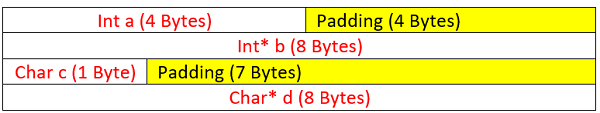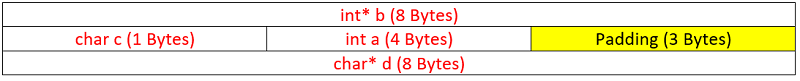# Size of struct in C | padding, alignment in struct

In this article, we are going to see what is the size of a struct in C? We will also learn what is padding, alignment provided by the compiler while defining memory for a struct.
Submitted by Radib Kar, on July 19, 2020

What we know is that size of a struct is the sum of all the data members. Like for the following struct,

```struct A{
int a;
int* b;
char c;
char *d;
};
```

Size of the struct should be sum of all the data member, which is: Size of int a+ size of int* b +size of char c+ size of char* d

```Now considering the 64-bit system,
Size of int is 4 Bytes
Size of character is 1 Byte
Size of any pointer type is 8 Bytes
(Pointer size doesn't depend on what kind of data type they are pointing too)

So the size of the struct should be: (4+8+1+8)=21 Bytes
```

Let's see what compiler is giving using the sizeof() operator.

```#include <stdio.h>

struct A {
int a;
int* b;
char c;
char* d;
};

int main()
{
struct A a;
printf("Size of struct A: %lu\n", sizeof(struct A));
printf("Size of object a: %lu\n", sizeof(a));
return 0;
}
```

Output:

```Size of struct A: 32
Size of object a: 32
```

Oops!! The output is 32 Bytes. How is that possible?

It seems like the compiler took maximum size out of the data type and assigned the same memory to all data types. Is it so?

Okay, it's quite like that, but not the same. Of course, the compiler adds padding and tries to align the data members. So for the above structure, the data alignment looks like below,Above is the alignment of the structure A, and that's why the size of the struct is 32 Bytes. Also, the object a of type struct A is 32 Bytes.

Now the question is how compiler adds padding and align? The method is compiler dependent and kind of greedy. It aligns till the boundary of maximum memory allocated. Here we find that max memory allocated is 8 Bytes, thus all the data members acquire 8 Bytes and the total size is 32 Bytes. Now the question is will it happen every time similarly?

Is it like the number of data members * max datatype size?

The answer is no. Check the following structure which has the same members but the ordering is different.

``` struct B{
int* b;
char c;
int a;
char *d;
};
```
```#include <stdio.h>

struct B {
int* b;
char c;
int a;
char* d;
};

int main()
{
struct B b;
printf("Size of struct B: %lu\n", sizeof(struct B));
printf("Size of object b: %lu\n", sizeof(b));
return 0;
}
```

Output:

```Size of struct B: 24
Size of object b: 24
```

In the above structure, we find that the size is 24 Bytes though the same data members have been used. This is due to the change in the order of the member declaration. In this case, the alignment and padding would be like below:Above is the alignment for structure B and that's why size is 24 Bytes, instead of 32. We saw that compiler keeps aligning greedily and that's why it aligned char c & int a in the same row. When it tried to align char* d, it could not as only 3 bytes were left. But instead of char*, if it was char only then it would have aligned in the same line.

So, I hope it's clear how compiler aligns a structure. A point to be noted is that compiler can't reorder the data members though it may have reduced size. Thus, struct A will have size 32 Bytes, not 24 Bytes.